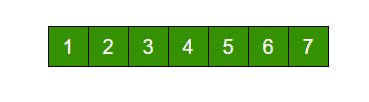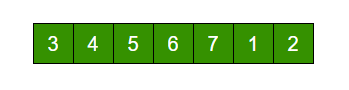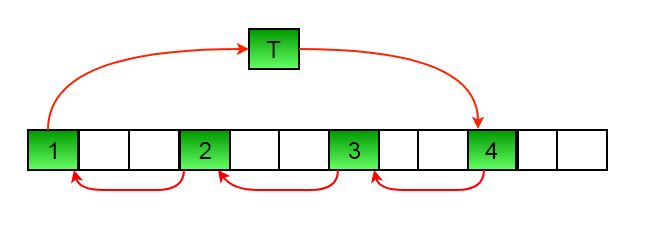Related Articles
Java Program For Array Rotation
• Last Updated : 03 Mar, 2021

Write a function rotate(ar[], d, n) that rotates arr[] of size n by d elements.Rotation of the above array by 2 will make arrayMETHOD 1 (Using temp array)

```Input arr[] = [1, 2, 3, 4, 5, 6, 7], d = 2, n =7
1) Store d elements in a temp array
temp[] = [1, 2]
2) Shift rest of the arr[]
arr[] = [3, 4, 5, 6, 7, 6, 7]
3) Store back the d elements
arr[] = [3, 4, 5, 6, 7, 1, 2]```

Time complexity : O(n)
Auxiliary Space : O(d)

METHOD 2 (Rotate one by one)

```leftRotate(arr[], d, n)
start
For i = 0 to i < d
Left rotate all elements of arr[] by one
end```

To rotate by one, store arr in a temporary variable temp, move arr to arr, arr to arr …and finally temp to arr[n-1]

Let us take the same example arr[] = [1, 2, 3, 4, 5, 6, 7], d = 2
Rotate arr[] by one 2 times
We get [2, 3, 4, 5, 6, 7, 1] after first rotation and [ 3, 4, 5, 6, 7, 1, 2] after second rotation.

## Java

 `class` `RotateArray ``{``    ``/*Function to left rotate arr[] of size n by d*/``    ``void` `leftRotate(``int` `arr[], ``int` `d, ``int` `n) ``    ``{``        ``int` `i;``        ``for` `(i = ``0``; i < d; i++)``            ``leftRotatebyOne(arr, n);``    ``}`` ` `    ``void` `leftRotatebyOne(``int` `arr[], ``int` `n) ``    ``{``        ``int` `i, temp;``        ``temp = arr[``0``];``        ``for` `(i = ``0``; i < n - ``1``; i++)``            ``arr[i] = arr[i + ``1``];``        ``arr[i] = temp;``    ``}`` ` `    ``/* utility function to print an array */``    ``void` `printArray(``int` `arr[], ``int` `size) ``    ``{``        ``int` `i;``        ``for` `(i = ``0``; i < size; i++)``            ``System.out.print(arr[i] + ``" "``);``    ``}`` ` `    ``// Driver program to test above functions``    ``public` `static` `void` `main(String[] args) ``    ``{``        ``RotateArray rotate = ``new` `RotateArray();``        ``int` `arr[] = {``1``, ``2``, ``3``, ``4``, ``5``, ``6``, ``7``};``        ``rotate.leftRotate(arr, ``2``, ``7``);``        ``rotate.printArray(arr, ``7``);``    ``}``}`` ` `// This code has been contributed by Mayank Jaiswal`

Output :

```3 4 5 6 7 1 2
```

Time complexity : O(n * d)
Auxiliary Space : O(1)
METHOD 3 (A Juggling Algorithm)
This is an extension of method 2. Instead of moving one by one, divide the array in different sets
where number of sets is equal to GCD of n and d and move the elements within sets.
If GCD is 1 as is for the above example array (n = 7 and d =2), then elements will be moved within one set only, we just start with temp = arr and keep moving arr[I+d] to arr[I] and finally store temp at the right place.

Here is an example for n =12 and d = 3. GCD is 3 and

```Let arr[] be {1, 2, 3, 4, 5, 6, 7, 8, 9, 10, 11, 12}

a)    Elements are first moved in first set – (See below diagram for this movement)arr[] after this step --> {4 2 3 7 5 6 10 8 9 1 11 12}

b)    Then in second set.
arr[] after this step --> {4 5 3 7 8 6 10 11 9 1 2 12}

c)    Finally in third set.
arr[] after this step --> {4 5 6 7 8 9 10 11 12 1 2 3}
```

## Java

 `class` `RotateArray ``{``    ``/*Function to left rotate arr[] of size n by d*/``    ``void` `leftRotate(``int` `arr[], ``int` `d, ``int` `n) ``    ``{``        ``int` `i, j, k, temp;``        ``for` `(i = ``0``; i < gcd(d, n); i++) ``        ``{``            ``/* move i-th values of blocks */``            ``temp = arr[i];``            ``j = i;``            ``while` `(``true``) ``            ``{``                ``k = j + d;``                ``if` `(k >= n) ``                    ``k = k - n;``                ``if` `(k == i) ``                    ``break``;``                ``arr[j] = arr[k];``                ``j = k;``            ``}``            ``arr[j] = temp;``        ``}``    ``}`` ` `    ``/*UTILITY FUNCTIONS*/``     ` `    ``/* function to print an array */``    ``void` `printArray(``int` `arr[], ``int` `size) ``    ``{``        ``int` `i;``        ``for` `(i = ``0``; i < size; i++)``            ``System.out.print(arr[i] + ``" "``);``    ``}`` ` `    ``/*Function to get gcd of a and b*/``    ``int` `gcd(``int` `a, ``int` `b) ``    ``{``        ``if` `(b == ``0``)``            ``return` `a;``        ``else``            ``return` `gcd(b, a % b);``    ``}`` ` `    ``// Driver program to test above functions``    ``public` `static` `void` `main(String[] args) {``        ``RotateArray rotate = ``new` `RotateArray();``        ``int` `arr[] = {``1``, ``2``, ``3``, ``4``, ``5``, ``6``, ``7``};``        ``rotate.leftRotate(arr, ``2``, ``7``);``        ``rotate.printArray(arr, ``7``);``    ``}``}`` ` `// This code has been contributed by Mayank Jaiswal`

Output :

```3 4 5 6 7 1 2
```

Time complexity : O(n)
Auxiliary Space : O(1)

Please refer complete article on Program for array rotation for more details!

Attention reader! Don’t stop learning now. Get hold of all the important DSA concepts with the DSA Self Paced Course at a student-friendly price and become industry ready.

My Personal Notes arrow_drop_up